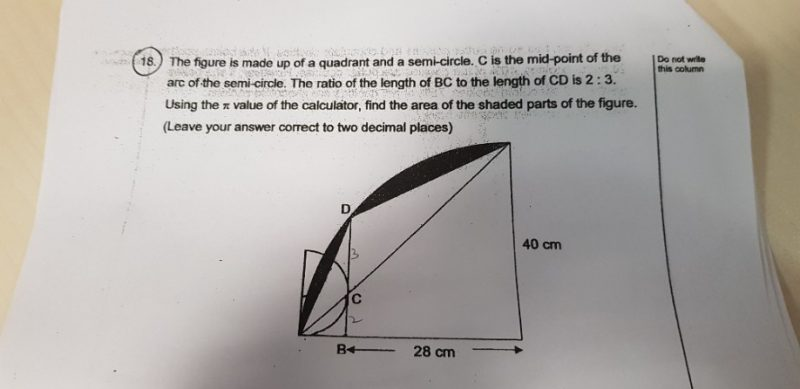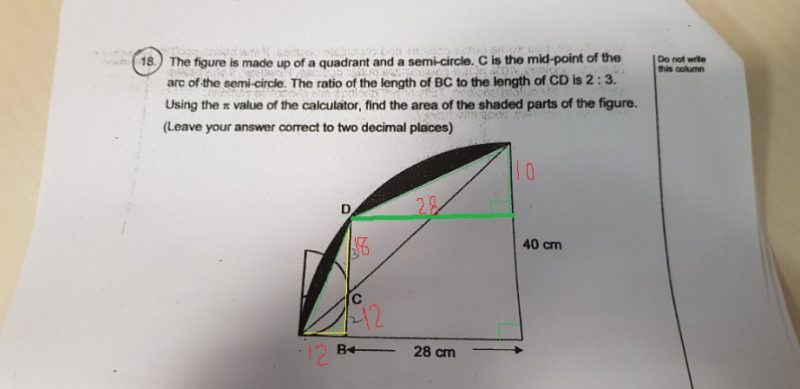# QuestionHello, can i get help for this question pls. ThanksIn my rusty memory, its Area of circle divide by four , that’s the total area of the sector, that is Pi R sq X theta by 360 = 3.141x40x40x(90/360) = 1256.6 sq cm
As its a part of the circle, the radius is same i.e. 40, remainder part of the radius from B to circumference would be 40-28 = 12 cm.
the smaller semicircle at bottom showing BC as tangent perpendicular to radius parallel, indicate BC would also be 12 cm. As CD is supposed to 3/2 of BC,CD will be 18 cms, making BD 30 CM
the area of 30×28 rectangle = 840 Sq cm ,
the area of remainder top triangle 1/2 base x ht = 1/2x28x10, = 140 Sq cm
the remainder bottom triangle 1/2 base x ht. = 1/2 30(BD) x 12. = 180 Sq cm
Removing these areas from Area of the square = 1256.60 – (840+140+180) = 96.6 Sq Cm

Again its from my old rusty memory of Math forumalae, so plz dont accept it without double checking yourself.
Get it double checked.

interesting problem though.

Thanks

0 Replies 0 Likes